# 13 Results

View
Selected filters:
• MCCRS.Math.Content.8.EE.C.8b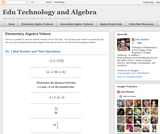Conditions of Use:
Remix and Share
Rating

Visually searchable database of Algebra 1 videos. Click on a problem and watch the solution on YouTube. Copy and paste this material into your CMS. Videos accompany the open Elementary Algebra textbook published by Flat World Knowledge.

Subject:
Algebra
Material Type:
Lecture
Lecture Notes
Provider:
Individual Authors
Provider Set:
Individual Authors
Author:
John Redden
04/29/2012Rating

This lesson unit is intended to help teachers assess how well students are able to create and solve linear equations. In particular, the lesson will help you identify and help students who have the following difficulties: solving equations with one variable and solving linear equations in more than one way.

Subject:
Algebra
Material Type:
Assessment
Lesson Plan
Provider:
Shell Center for Mathematical Education
U.C. Berkeley
Provider Set:
Mathematics Assessment Project (MAP)
04/26/2013Rating

This lesson unit is intended to help teachers assess how well students are able to classify solutions to a pair of linear equations by considering their graphical representations. In particular, this unit aims to help teachers identify and assist students who have difficulties in: using substitution to complete a table of values for a linear equation; identifying a linear equation from a given table of values; and graphing and solving linear equations.

Subject:
Algebra
Material Type:
Assessment
Lesson Plan
Provider:
Shell Center for Mathematical Education
U.C. Berkeley
Provider Set:
Mathematics Assessment Project (MAP)
04/26/2013Conditions of Use:
Rating

The foundation of this lesson is constructing, communicating, and evaluating student-generated tables while making comparisons between three different financial plans. Students are given three different DVD rental plans and asked to analyze each one to see if they could determine when the 3 different DVD plans cost the same amount of money, if ever. (7th/8th Grade Math)

Subject:
Algebra
Material Type:
Activity/Lab
Lecture
Lesson Plan
Teaching/Learning Strategy
Provider:
Noyce Foundation
Provider Set:
Inside Mathematics
Author:
Dimas, Cecilio
11/30/2011Rating

This lesson unit is intended to help teachers assess how well students are able to: estimate lengths of everyday objects; convert between decimal and scientific notation; and make comparisons of the size of numbers expressed in both decimal and scientific notation.

Subject:
Mathematics
Material Type:
Assessment
Lesson Plan
Provider:
Shell Center for Mathematical Education
U.C. Berkeley
Provider Set:
Mathematics Assessment Project (MAP)
04/26/2013Rating

This lesson unit is intended to help you assess how well students working with square numbers are able to: choose an appropriate, systematic way to collect and organize data, examining the data for patterns; describe and explain findings clearly and effectively; generalize using numerical, geometrical, graphical and/or algebraic structure; and explain why certain results are possible/impossible, moving towards a proof.

Subject:
Algebra
Geometry
Measurement and Data
Material Type:
Assessment
Lesson Plan
Provider:
Shell Center for Mathematical Education
U.C. Berkeley
Provider Set:
Mathematics Assessment Project (MAP)
04/26/2013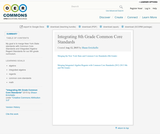Conditions of Use:
No Strings Attached
Rating

My goal is to merge New York State standards with Common Core Standards and Integrated Algebra Regent Standards for our 8th grade curriculum.

Subject:
Mathematics
Material Type:
Full Course
Lesson Plan
Syllabus
Author:
Shaun Errichiello
01/28/2016Rating

This lesson unit is intended to help teahcers assess how well students are able to interpret speed as the slope of a linear graph and translate between the equation of a line and its graphical representation.

Subject:
Algebra
Material Type:
Assessment
Lesson Plan
Provider:
Shell Center for Mathematical Education
U.C. Berkeley
Provider Set:
Mathematics Assessment Project (MAP)
04/26/2013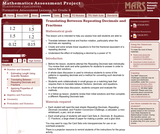Rating

This lesson unit is intended to help teachers assess how well students are able to: translate between decimal and fraction notation, particularly when the decimals are repeating; create and solve simple linear equations to find the fractional equivalent of a repeating decimal; and understand the effect of multiplying a decimal by a power of 10.

Subject:
Algebra
Ratios and Proportions
Material Type:
Assessment
Lesson Plan
Provider:
Shell Center for Mathematical Education
U.C. Berkeley
Provider Set:
Mathematics Assessment Project (MAP)
04/26/2013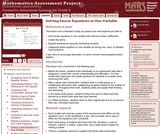Rating

This lesson unit is intended to help teachers assess how well students are able to: solve linear equations in one variable with rational number coefficients; collect like terms; expand expressions using the distributive property; and categorize linear equations in one variable as having one, none, or infinitely many solutions. It also aims to encourage discussion on some common misconceptions about algebra.

Subject:
Algebra
Material Type:
Assessment
Lesson Plan
Provider:
Shell Center for Mathematical Education
U.C. Berkeley
Provider Set:
Mathematics Assessment Project (MAP)
04/26/2013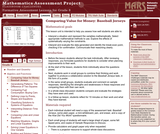Rating

This lesson unit is intended to help teachers assess how well students are able to: interpret a situation and represent the variables mathematically; select appropriate mathematical methods to use; explore the effects of systematically varying the constraints; interpret and evaluate the data generated and identify the break-even point, checking it for confirmation; and communicate their reasoning clearly.

Subject:
Mathematics
Material Type:
Assessment
Lesson Plan
Provider:
Shell Center for Mathematical Education
U.C. Berkeley
Provider Set:
Mathematics Assessment Project (MAP)
04/26/2013Conditions of Use:
No Strings Attached
Rating

This module contains the a systems of linear equations from Elementary Algebra by Denny Burzynski and Wade Ellis, Jr.

Subject:
Algebra
Material Type:
Provider:
Rice University
Provider Set:
Connexions
Author:
Denny Burzynski
02/16/2011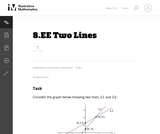Conditions of Use:
No Strings Attached
Rating

In this task, we are given the graph of two lines including the coordinates of the intersection point and the coordinates of the two vertical intercepts, and are asked for the corresponding equations of the lines. It is a very straightforward task that connects graphs and equations and solutions and intersection points.

Subject:
Mathematics
Material Type:
Activity/Lab
Provider:
Illustrative Mathematics
Provider Set:
Illustrative Mathematics
Author:
Illustrative Mathematics